# OECD Household disposable income data analysis 6 - Time-Series Data Regression using RPhoto by Jeremy Bishop on Unsplash

This post is following of above post.
In this post, I will do time-series data regression using R.

Firstly, I converted avg_gr_us in 10000 value.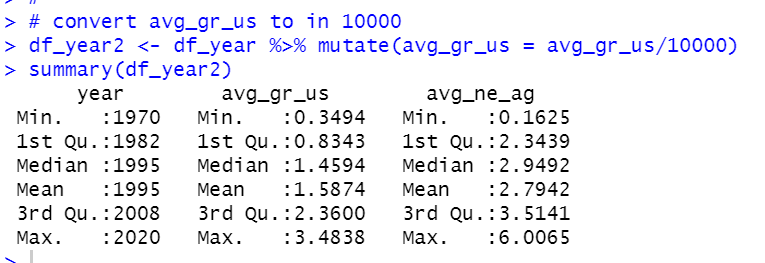Then, I converted df_year2 data frame to ts object.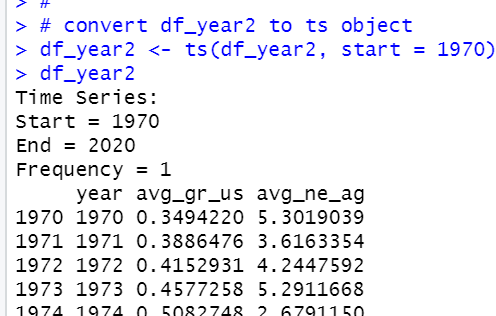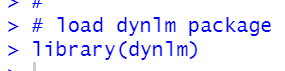Let's begin with "static model"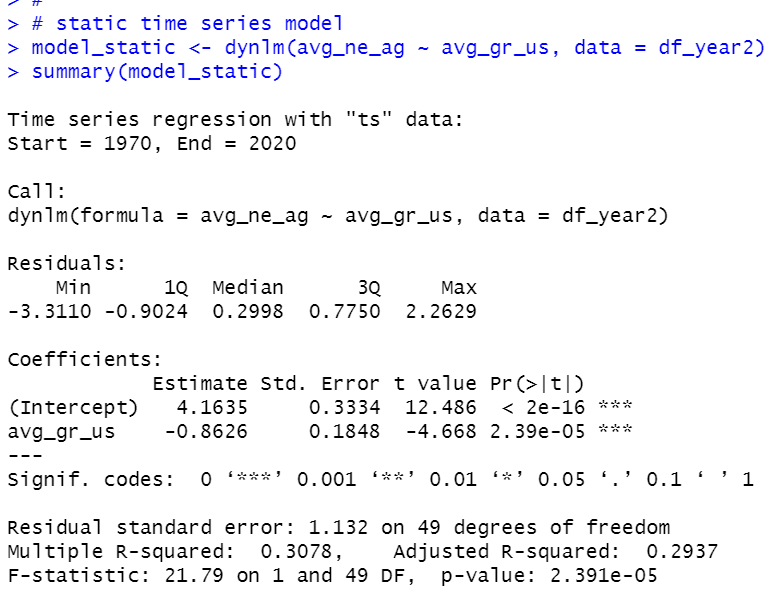We see avg_gr_us and avg_ne_ag are negative correlation.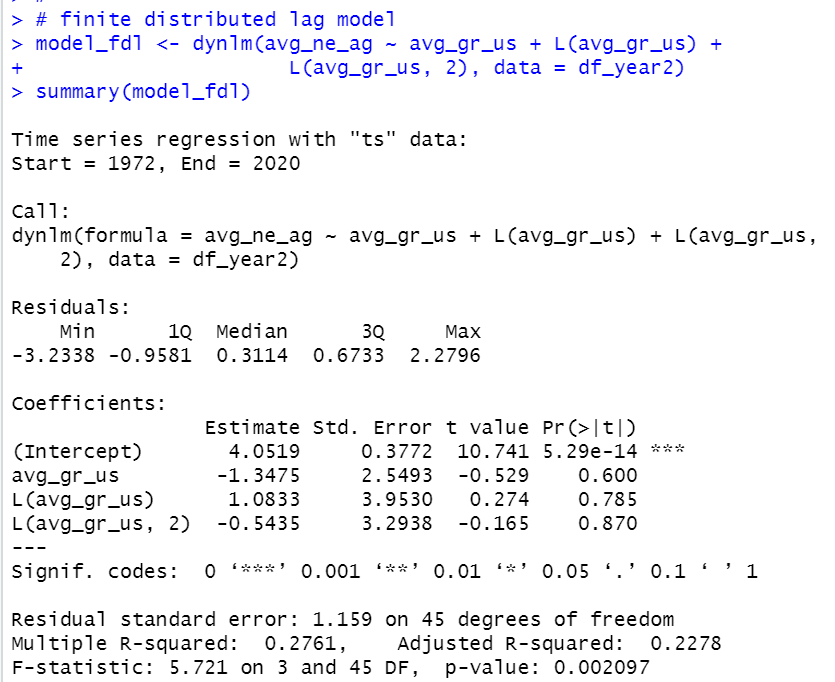Aggregate coefficeitns are -1.3475 + 1.0833 - 0.5435 = -0.8077.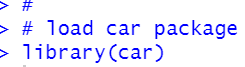Then, I do F test to test H0: all "avg_gr_us" coefficients are = 0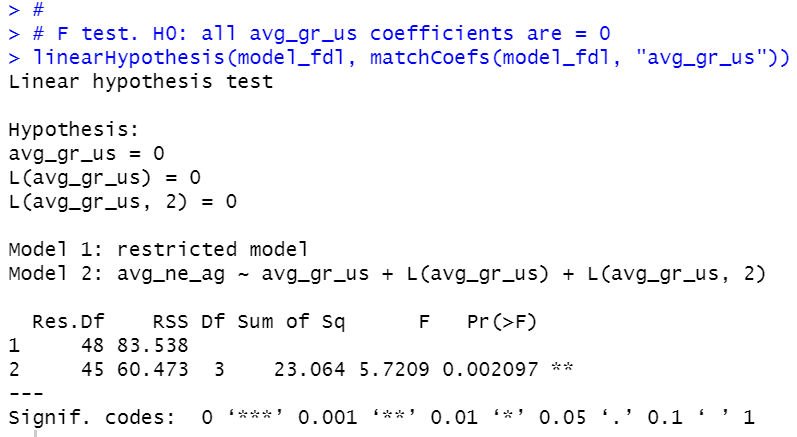p-value is 0.002097, so I reject H0.

Then, I made trend only model.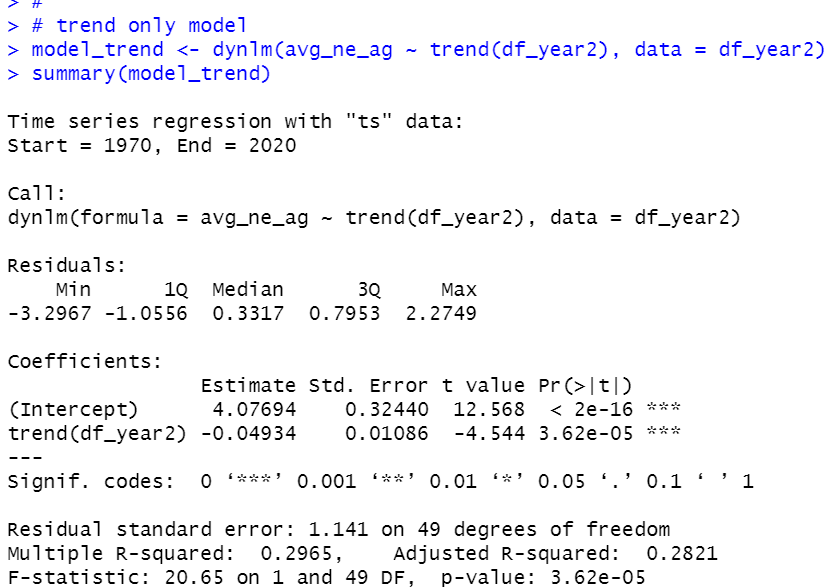We see trend coefficient is statistically significant.

Then, I made trend + avg_gr_us model.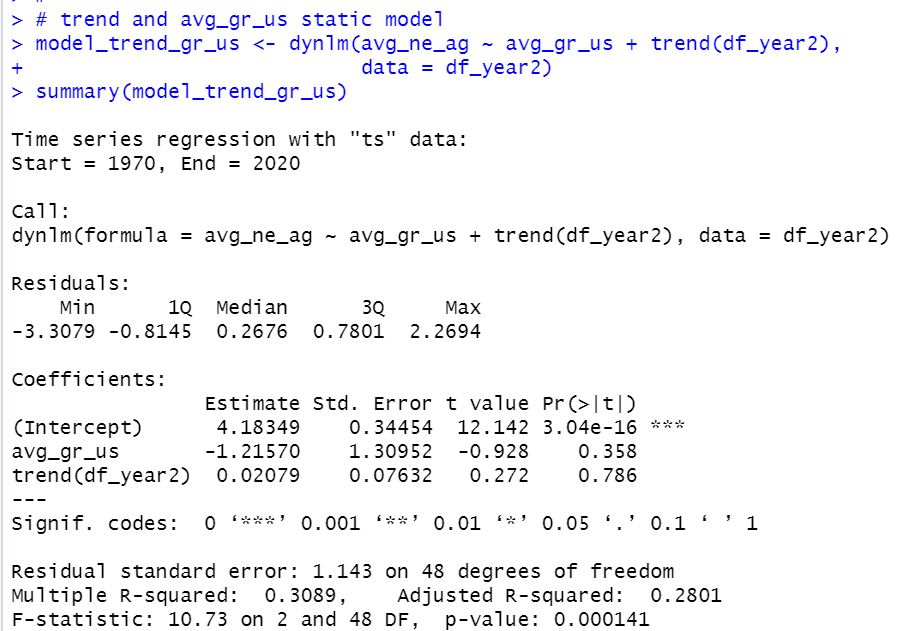p-value is 0.000141 so this model is statistically significant.

That}s it. Thank you!

Next post is